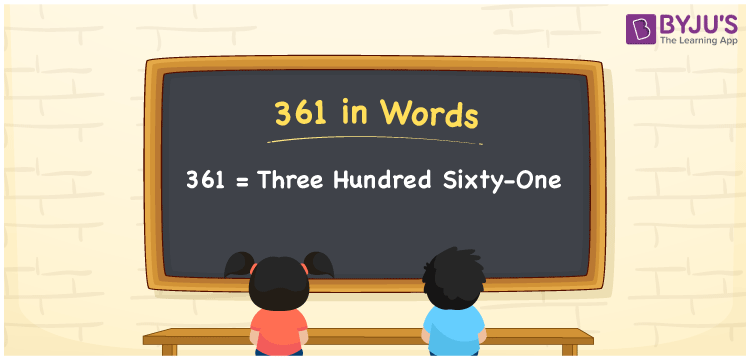# 361 in Words

361 in words is written as Three hundred sixty-one. In both the International System of Numerals and the Indian System of Numerals, 361 is written as Three hundred sixty-one. The number 361 is a Cardinal Number as it represents some quantity. For example, the “cost of the t-shirt is 361 rupees”.

 361 in Words Three hundred sixty-one Three hundred sixty-one in Number 361

## 361 in English Words

We write 361 in English Words using the letters of the English alphabet. Therefore, we read 361 in English as “Three hundred sixty-one”.## How to Write 361 in Words?

To write 361 in words, we shall use the place value chart. In the place value chart, write 3 in the hundreds, 6 in the tens, and 1 in the ones, respectively. Now let us make a place value chart to write the number 361 in words.

 Hundreds Tens Ones 3 6 1

Thus, we can write the expanded form as

3 × Hundred + 6 × Ten + 1 × One

= 3 × 100 + 6 × 10 + 1 × 1

= 300 + 60 + 1

= 361

= Three hundred sixty-one.

361 is a natural number, the successor of 360 and the predecessor of 362.

361 in words – Three hundred sixty-one

• Is 361 an odd number? – Yes
• Is 361 an even number? – No
• Is 361 a perfect square number? – No
• Is 361 a perfect cube number? – No
• Is 361 a prime number? – No
• Is 361 a composite number? – Yes

## Frequently Asked Questions on 361 in Words

Q1

### How to write 361 in words?

361 in words is written as Three hundred sixty-one.
Q2

### How to write 361 in the International and Indian System of Numerals?

In both, the system of numerals, 361 in words, is written as Three hundred sixty-one.
Q3

### What is the successor of 361?

The successor of 361 is 362.